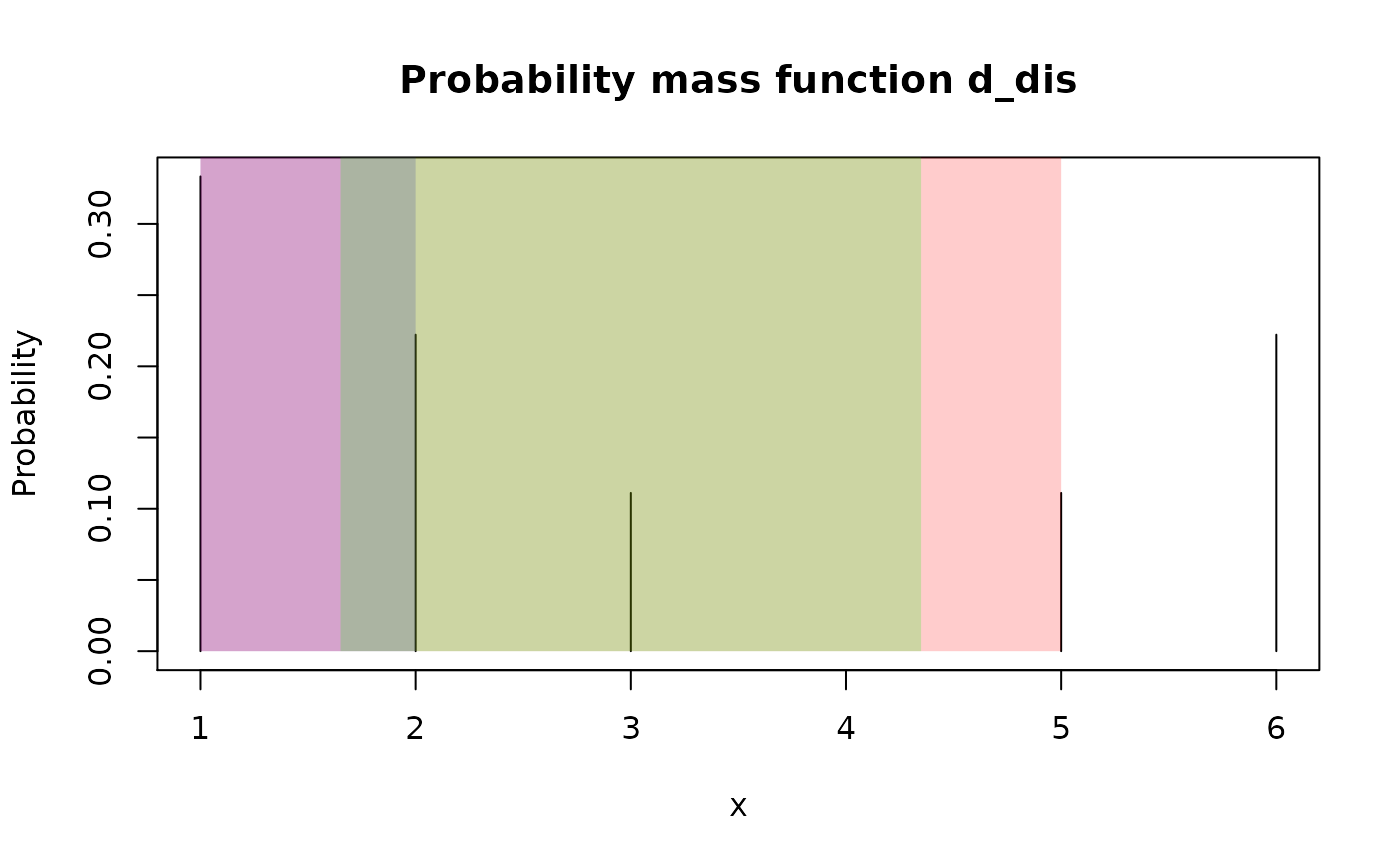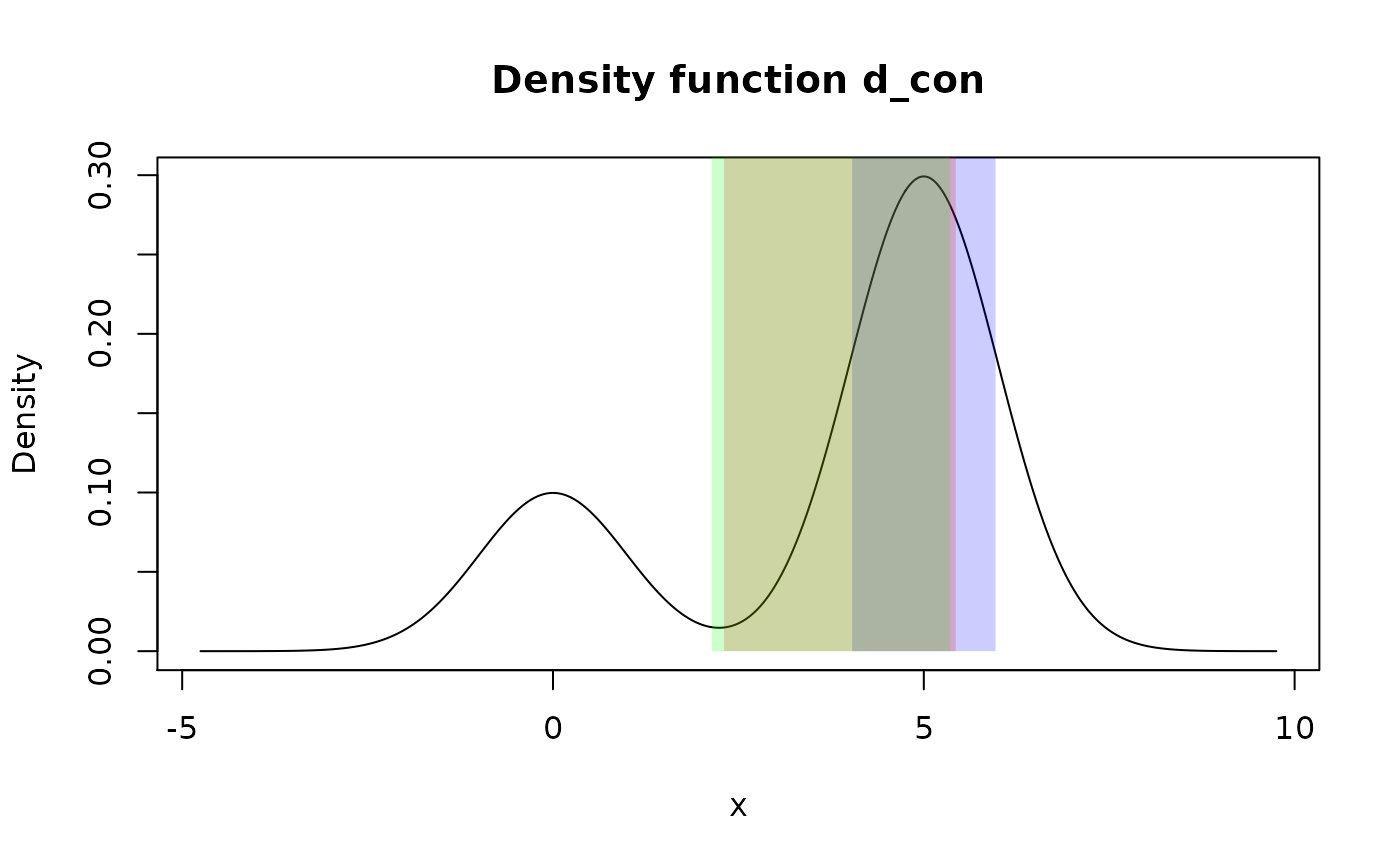These functions summarize distribution with one interval based on method of choice.

summ_interval(f, level = 0.95, method = "minwidth", n_grid = 10001)

## Arguments

f A pdqr-function representing distribution. A number between 0 and 1 representing a coverage degree of interval. Interpretation depends on method but the bigger is number, the wider is interval. Method of interval computation. Should be on of "minwidth", "percentile", "sigma". Number of grid elements to be used for "minwidth" method (see Details).

## Value

A region with one row. That is a data frame with one row and the following columns:

• left <dbl> : Left end of interval.

• right <dbl> : Right end of interval.

To return a simple numeric vector, call unlist() on summ_interval()'s output (see Examples).

## Details

Method "minwidth" searches for an interval with total probability of level that has minimum width. This is done with grid search: n_grid possible intervals with level total probability are computed and the one with minimum width is returned (if there are several, the one with the smallest left end). Left ends of computed set of intervals are created as a grid from 0 to 1-level quantiles with n_grid number of elements. Right ends are computed so that intervals have level total probability.

Method "percentile" returns an interval with edges being 0.5*(1-level) and 1 - 0.5*(1-level) quantiles. Output has total probability equal to level.

Method "sigma" computes an interval symmetrically centered at mean of distribution. Left and right edges are distant from center by the amount of standard deviation multiplied by level's critical value. Critical value is computed using normal distribution as qnorm(1 - 0.5*(1-level)), which corresponds to a way of computing sample confidence interval with known standard deviation. The final output interval is possibly cut so that not to be out of f's support.

Note that supported methods correspond to different ways of computing distribution's center. This idea is supported by the fact that when level is 0, "minwidth" method returns zero width interval at distribution's global mode, "percentile" method - median, "sigma" - mean.

summ_hdr() for computing of Highest Density Region, which can summarize distribution with multiple intervals.

region_*() family of functions for working with summ_interval() output.

Other summary functions: summ_center(), summ_classmetric(), summ_distance(), summ_entropy(), summ_hdr(), summ_moment(), summ_order(), summ_prob_true(), summ_pval(), summ_quantile(), summ_roc(), summ_separation(), summ_spread()

## Examples

# Type "discrete"
d_dis <- new_d(data.frame(x = 1:6, prob = c(3:1, 0:2) / 9), "discrete")
summ_interval(d_dis, level = 0.5, method = "minwidth")
#>   left right
#> 1    1     2summ_interval(d_dis, level = 0.5, method = "percentile")
#>   left right
#> 1    1     5summ_interval(d_dis, level = 0.5, method = "sigma")
#>      left   right
#> 1 1.65102 4.34898
## Visual difference between methods
plot(d_dis)
region_draw(summ_interval(d_dis, 0.5, method = "minwidth"), col = "blue")
region_draw(summ_interval(d_dis, 0.5, method = "percentile"), col = "red")
region_draw(summ_interval(d_dis, 0.5, method = "sigma"), col = "green")# Type "continuous"
d_con <- form_mix(
list(as_d(dnorm), as_d(dnorm, mean = 5)),
weights = c(0.25, 0.75)
)
summ_interval(d_con, level = 0.5, method = "minwidth")
#>       left    right
#> 1 4.032616 5.967419summ_interval(d_con, level = 0.5, method = "percentile")
#>       left    right
#> 1 2.305452 5.430726summ_interval(d_con, level = 0.5, method = "sigma")
#>       left    right
#> 1 2.141451 5.358549
## Visual difference between methods
plot(d_con)
region_draw(summ_interval(d_con, 0.5, method = "minwidth"), col = "blue")
region_draw(summ_interval(d_con, 0.5, method = "percentile"), col = "red")
region_draw(summ_interval(d_con, 0.5, method = "sigma"), col = "green")# Output interval is always inside input's support. Formally, next code
# should return interval from -Inf to Inf, but output is cut to be inside
# support.
summ_interval(d_con, level = 1, method = "sigma")
#>        left    right
#> 1 -4.753424 9.753424
# To get vector output, use unlist()
unlist(summ_interval(d_con))
#>      left     right
#> -1.199428  6.906784